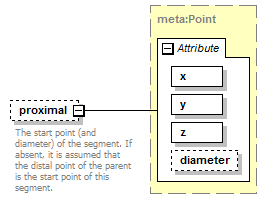# proximal

 `The start point (and diameter) of the segment. If absent, it is assumed that the distal point of the parent is the start point of this segment.`

## Model## Attributes

QName Type Fixed Default Use Inheritable Annotation
diameter xs:double optional
x xs:double required
y xs:double required
z xs:double required

## Source

 ``` The start point (and diameter) of the segment. If absent, it is assumed that the distal point of the parent is the start point of this segment. ```